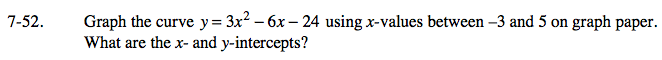### Home > AC > Chapter 7 > Lesson 7.1.5 > Problem7-52

7-52.

Graph the curve y = 3x2 − 6x − 24 using x-values between −3 and 5 on graph paper. What are the x- and y-intercepts? Homework Help ✎Use a table to find points on the graph by substituting values between −3 and 5 into
the equation, then graph the curve and find the intercepts.